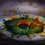# $\color{#D61F06}{\textbf{FullStop}}$ to the disputes- JOMO 7

First of all, when at first the question file of $\textbf{JOMO 7}$ was uploaded, there was a little flaw in question $9$ (short) , and so we uploaded the new file with an addition in the question (length of AC)

But later, when the results were out and solution file was uploaded, the solution was as per the previous (wrong) version of the question, so results will be posted once again.

To put a fullstop to all the disputes and clarifications, I am writing this note.

Question (JOMO 7, short 9) :- In $\triangle ABC$ ,$I$ is the incenter, and $AI,BI,CI$ meet the sides $BC,AC,AB$ at points $D,E,F$ respectively. $AB=20 , BC=14 , AC=\dfrac{438}{53}$. Then the ratio $\dfrac{ID}{IF}$ can be written as $\dfrac{a}{b}$ where $b$ and $a$ are coprime positive integers, find $a+b$

Solution :-img

For convenience, $AB=c,BC=a,AC=b$

We will use the angle bisector property in $\triangle ABC$ ,

See that $\dfrac{DC}{BD} = \dfrac{b}{c} \implies \dfrac{BD}{BC}=\dfrac{c}{b+c}$

Thus we have $BD=\dfrac{ac}{b+c}$

Now, we use angle bisector property for $\triangle BAD$ ,

$\dfrac{BD}{AB} = \dfrac{ID}{AI} = \dfrac{\frac{ac}{b+c}}{c} = \dfrac{a}{b+c}$

Thus we get $\dfrac{ID}{AD} = \dfrac{a}{a+b+c}$

Now, $AD = \dfrac{\sqrt{bc}}{b+c} \sqrt{(b+c)^2-a^2}$ (length of the angle bisector)

So we get the value of $ID= \dfrac{a}{a+b+c}\times \dfrac{\sqrt{bc}}{b+c} \sqrt{(b+c)^2-a^2}$

Similarly, we get $IF= \dfrac{c}{a+b+c} \times \dfrac{\sqrt{ab}}{a+b} \sqrt{(b+a)^2-c^2}$

Then , after taking their ratio, answer comes as

$\dfrac{ID}{IF} = \dfrac{(b+a) \sqrt{[(b+c)^2-a^2]a}}{(b+c) \sqrt{[(b+a)^2-c^2]c}}$

$\dfrac{ID}{IF}= \dfrac{(b+a) \sqrt{(b+c-a)a}}{(b+c) \sqrt{(a+b-c)c}}$

And, finally, putting values

$\dfrac{ID}{IF} = \dfrac{(b+14)\sqrt{(b+6)14}}{(b+20)\sqrt{(b-6)20}}$

And when you put $b=\dfrac{438}{53}$, answer is $\dfrac{177}{107}$, hence the asked value is $177+107=\boxed{284}$.6 years, 9 months ago

This discussion board is a place to discuss our Daily Challenges and the math and science related to those challenges. Explanations are more than just a solution — they should explain the steps and thinking strategies that you used to obtain the solution. Comments should further the discussion of math and science.

When posting on Brilliant:

• Use the emojis to react to an explanation, whether you're congratulating a job well done , or just really confused .
• Ask specific questions about the challenge or the steps in somebody's explanation. Well-posed questions can add a lot to the discussion, but posting "I don't understand!" doesn't help anyone.
• Try to contribute something new to the discussion, whether it is an extension, generalization or other idea related to the challenge.

MarkdownAppears as
*italics* or _italics_ italics
**bold** or __bold__ bold
- bulleted- list
• bulleted
• list
1. numbered2. list
1. numbered
2. list
Note: you must add a full line of space before and after lists for them to show up correctly
paragraph 1paragraph 2

paragraph 1

paragraph 2

[example link](https://brilliant.org)example link
> This is a quote
This is a quote
    # I indented these lines
# 4 spaces, and now they show
# up as a code block.

print "hello world"
# I indented these lines
# 4 spaces, and now they show
# up as a code block.

print "hello world"
MathAppears as
Remember to wrap math in $$ ... $$ or $ ... $ to ensure proper formatting.
2 \times 3 $2 \times 3$
2^{34} $2^{34}$
a_{i-1} $a_{i-1}$
\frac{2}{3} $\frac{2}{3}$
\sqrt{2} $\sqrt{2}$
\sum_{i=1}^3 $\sum_{i=1}^3$
\sin \theta $\sin \theta$
\boxed{123} $\boxed{123}$

Sort by:

How can you derive angle-bisector theorem?

- 6 years, 9 months ago

I won't write it all here.... see it here , i know it is clear enough... ;)

- 6 years, 9 months ago

Thanks!! Can't we use tangent-secant theorem here? The answer will not be satisfactory, BTW!!

- 6 years, 9 months ago

Good.use what you think is more elegant ;) gud luck problem solving....

- 6 years, 9 months ago

@Aditya Raut Since u' luv b'uutifiing Brilliant, u' may like ter b'uutify yurr image as well. Try givin' yurr image the same background as Brilliant pages 'ave, 'twill look b'uutifull.

- 6 years, 9 months ago

And lol, what a new invention of language !

- 6 years, 9 months ago

Wait wait wait.... What exactly ? This image is an HD one, even my fb and gmail pro pic, it's cool already! Why make it brilliant color ?Img

(If you wanna see actual size,the $\color{#D61F06}{\textbf{bigger}}$ one it's here )

- 6 years, 9 months ago

Noah. 'was talkin' abou' the image in 'dis post, the bagg-round 'iffers from the Brilliant bagg-round.

- 6 years, 9 months ago

I made that diagram in paint, so i didn't choose it that way... anyway, no matter, it doesn't make a big difference.

- 6 years, 9 months ago

Thanks, but don't you think you should instead mail it to all the JOMO users, since they're all not necessarily on Brilliant.

- 6 years, 9 months ago

We did that too, and this one is for making sure no more disputes come to us :P a fullstop

- 6 years, 9 months ago